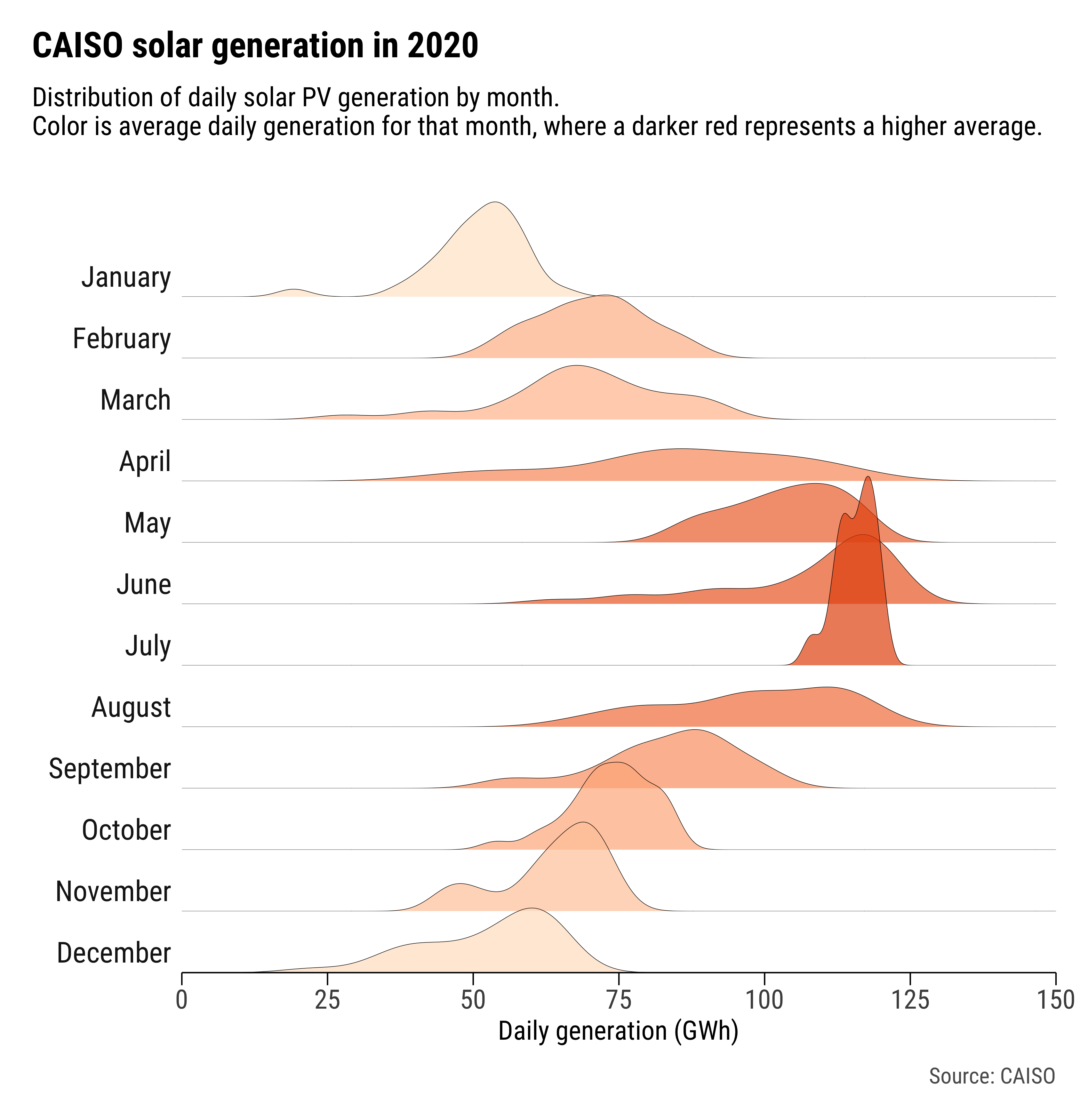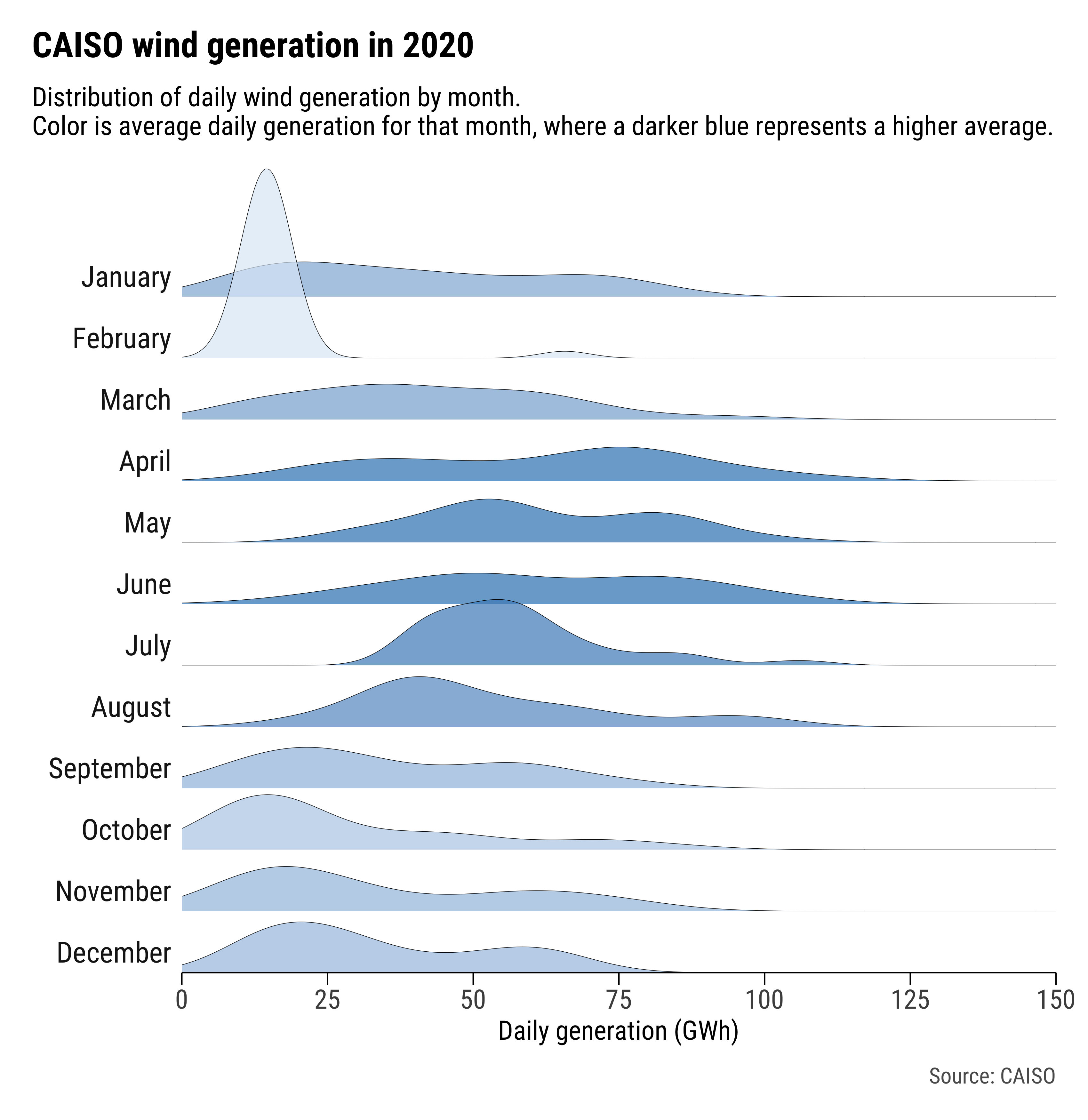# CAISO 2020 solar and wind generation ridgeline plotsVisualizing the distribution of renewable generation in CAISO by month 15 March 2021

## Intro

There are two things I’ve been meaning to learn how to do:

1. Access CAISO data
2. Make ridgeline plots

So I spent this weekend trying to do both. The end result? Ridgeline plots showing the distribution of solar and wind generation within the California Independent System Operator (CAISO) in 2020 across different months.

The figure below shows the result for solar PV generation. The colors correspond to the mean of daily generation values in each month. So, the darker the red color, the higher the average daily generation within that respective month.I made the same plot for wind generation in CAISO as well:Continue reading for how I collected the data and created the plots.

## The data

Accessing CAISO’s data has always intimidated me – it’s probably due to the difficulty I experience trying to navigate the OASIS platform. This weekend, I was doing a quick Google search of CAISO data and came across PyISO, a Python package that allows users to pull data from various balancing authorities. I didn’t actually install or try PyISO, but I did go through the code for their CAISO module. It appears PyISO pulls CAISO’s renewable electricity generation data from CAISO’s Daily Renewables Watch reports, which at the time I did not know about. A quick search brought me to this page. The Daily Renewables Watch reports can be accessed (and saved) as .txt files with the same URL format, with the only difference being the date. As an example, here’s a link to March 12, 2021’s report.

So, it seemed like if I wanted renewable generation data for all of 2020, I could loop through the Daily Renewables Watch report URLs for each day of the year and download the data. I created a loop that goes through each day between two specified dates, reads in the Daily Renewables Watch report for that date as a pandas dataframe, then appends the dataframe into a list of dataframes. After the loop is complete, the list of dataframes is concatenated together into a single dataframe.

``````import pandas as pd
from datetime import date, datetime, timedelta
import os

# specify start and end dates -------
start_date = '2020-01-01'
end_date = '2020-12-31'

# get dates between specified start and end dates -----
dates = pd.date_range(start_date, end_date, freq='d')

# base url of hourly breakdown reports -----
base_url = 'http://content.caiso.com/green/renewrpt'

# loop through dates to access URLs for all dates of hourly breakdown reports -------
print('Gathering data...')
appended_dfs = []
for i in range(0, dates.size):
'''
for each date, add the date to the base url and complete the url to get the needed url to access the data.
then, read each report in as a pandas dataframe
each dataframe is appended to the appended_dfs list
'''

# get url for each date
get_url = os.path.join(base_url, datetime.strftime(dates[i], '%Y%m%d') + '_DailyRenewablesWatch.txt')

# read in specified columns (the tab delimited data is weird, and the header columns don't match the following rows)

# rename columns
df.columns = ['Hour', 'GEOTHERMAL', 'BIOMASS', 'BIOGAS', 'SMALL HYDRO', 'WIND TOTAL', 'SOLAR PV', 'SOLAR THERMAL']

# add column for the date
df[['date']] = dates[i]

# reorder columns
df = df[['date', 'Hour',
'GEOTHERMAL', 'BIOMASS', 'BIOGAS', 'SMALL HYDRO',
'WIND TOTAL', 'SOLAR PV', 'SOLAR THERMAL']]

# append current date's dataframe to list of dataframes
appended_dfs.append(df)

# concat the list of dataframes into one dataframe -----
df_caiso = pd.concat(appended_dfs, axis=0, sort=False)
``````

To get the total daily generation from each fuel source, I simply aggregated the values of all the fuel columns to the date-level.

``````# rename columns ------
df_caiso.columns= df_caiso.columns.str.lower()
df_caiso.columns = df_caiso.columns.str.replace(' ', '_')

# convert columns to numeric -------
cols = ['geothermal', 'biomass', 'biogas', 'small_hydro', 'wind_total', 'solar_pv', 'solar_thermal']
df_caiso[cols] = df_caiso[cols].apply(pd.to_numeric, errors='coerce')

# find daily total generation of each renewable source ------
print('Data collection complete. Now processing data...')

df_daily = df_caiso[['date', 'geothermal', 'biomass', 'biogas', 'small_hydro',
'wind_total', 'solar_pv', 'solar_thermal']].groupby(['date']).sum().reset_index()
``````

The complete script to do all of this can be found in this repo, under scripts > `get-caiso-data.py`. The script outputs two csv files: one for all of the hourly generation combined together, and one for the daily aggregation. You can specify the dates of reports you want to pull from by specifying the `start_date` and `end_date` variables. The data from January 1, 2020 to December 31, 2020 that I used for this post is already included in the repo, under the data folder.

## The plots

I originally wanted to make the ridgeline plots using the Altair package in Python (like the example here). I managed to get a plot that was something along the lines of what I wanted using Altair, but I couldn’t save a high resolution version of the figure (seems to be an issue right now with `altair_saver` – once the issue is fixed, I’ll be excited to revisit the package).

So, I just decided to revert back to plotting in trusty ggplot2 (R). One day I will force myself to be more comfortable with using the `seaborn` package in Python, but Saturday, March 13, 2021 was not that day.

In terms of preparing the data for plotting, I didn’t have to do much. The only things I needed to do, that I didn’t do in the Python script, were:

1. Add a column for the month
2. Calculate the average daily generation for each month (for each fuel type).

Those are both easy to do in R:

``````# inputs ------------

data_file = 'caiso_renewables_daily_2020-01-01_2020-12-31.csv'

library(data.table)
library(ggplot2)
library(hrbrthemes)
library(extrafont)

# create column with month names ------

dt_caiso[, month := format(date, '%B')]

# find the average daily generation -------

cols = c('geothermal', 'biomass', 'biogas', 'small_hydro', 'wind_total', 'solar_pv', 'solar_thermal')
dt_caiso[, paste0(cols, "_mean") := lapply(.SD, mean), .SDcols = cols, by = .(month)]

# convert integer columns to numeric ------

dt_caiso[, solar_pv := as.numeric(as.character(solar_pv))]

# reorder month levels -----

dt_caiso[, month := factor(month, levels = c('January', 'February', 'March', 'April', 'May', 'June', 'July',
'August', 'September', 'October', 'November', 'December'))]
``````

As for the actual plotting, the ridgeline plots are essentially facted density plots where the y-axes have been removed, and the facets have been pushed closer together. I used the one produced by Bob Rudis on his blog as a guide (also his `hrbrthemes` package is one I constantly use when plotting to make my figures look nice). To create the wind generation ridgeline plot above, I did the following:

``````# plot theme -------

theme_line = theme_ipsum(base_family = 'Roboto Condensed',
grid = '',
plot_title_size = 16,
subtitle_size = 12,
axis_title_just = 'center',
axis_title_size = 12,
axis_text_size = 12,
strip_text_size = 13)  +
theme(plot.title = element_text(hjust = 0, face = 'bold'),
plot.title.position = 'plot',
plot.subtitle = element_text(hjust = 0),
plot.caption = element_text(size = 10, color = '#5c5c5c', face = 'plain'),
plot.margin = unit(c(1,1,1,1), 'lines'),
axis.line.x = element_line(color = 'black', size = 0.3),
axis.ticks.x = element_line(color = 'black', size = 0.3),
axis.ticks.length.x = unit(0.2, 'cm'),
axis.text.x = element_text(margin = margin(2, 0, 0, 0))) +
theme(strip.text.y.left = element_text(angle = 0, hjust = 1, vjust = 0)) +
theme(panel.spacing.y = unit(-4, 'lines')) +
theme(axis.text.y = element_blank()) +
theme(legend.position = 'none') +
theme(plot.subtitle = element_text(margin = margin(0,0,-15,0)))

# plot: solar pv -------

fig_solar = ggplot(dt_caiso, aes(x = solar_pv/1e3, fill = solar_pv_mean)) +
geom_density(alpha = 0.7, color = 'black', lwd = 0.1) +
labs(title = 'CAISO solar generation in 2020',
subtitle = 'Distribution of daily solar PV generation by month. \nColor is average daily generation for that month, where a darker red represents a higher average.',
caption = 'Source: CAISO',
x = 'Daily generation (GWh)',
y = NULL) +
facet_wrap(~month, ncol = 1, strip.position = "left", dir = "v") +
scale_fill_gradient(low = '#fee6ce', high = '#e6550d') +
scale_x_continuous(expand = c(0,0), limits = c(0, 150), breaks = seq(0, 150, 25)) +
scale_y_continuous(expand = c(0,0)) +
theme_line
``````

Then, I created a similar plot, but for wind generation instead:

``````fig_wind = ggplot(dt_caiso, aes(x = wind_total/1e3, fill = wind_total_mean)) +
geom_density(alpha = 0.7, color = 'black', lwd = 0.1) +
labs(title = 'CAISO wind generation in 2020',
subtitle = 'Distribution of daily wind generation by month. \nColor is average daily generation for that month, where a darker blue represents a higher average.',
caption = 'Source: CAISO',
x = 'Daily generation (GWh)',
y = NULL) +
facet_wrap(~month, ncol = 1, strip.position = "left", dir = "v") +
scale_fill_gradient(low = '#deebf7', high = '#3182bd') +
scale_x_continuous(expand = c(0,0), limits = c(0, 150), breaks = seq(0, 150, 25)) +
scale_y_continuous(expand = c(0,0)) +
theme_line
``````

The full R script to create the plots (using the aggregated daily csv file from the `get-caiso-data.py` script as an input) can be found in the repo, under scripts > `plot-caiso-data.R`.– All of the scripts needed to gather the CAISO data and create the ridgeline plots are located in this Github repository. The repo also includes the CSV files and figure (PDF and PNG) files created by the scripts.

*****